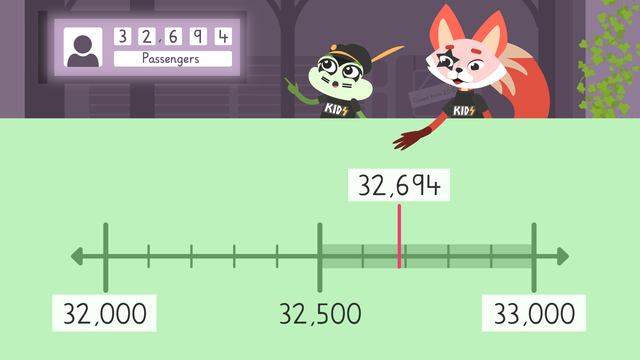# Rounding Multi-Digit Numbers on a Number LineRating

Ø 5.0 / 1 ratings

The authorsTeam Digital

## Basics on the topicRounding Multi-Digit Numbers on a Number Line

### Content

#### In this Rounding on a Number Line 4th Grade Video

Sklyar and Henry are at the train station waiting for the train to take them to see their favorite band, KIDS. Henry and Sklyar notice the fast-moving numbers of the passenger counter and can’t keep their eyes off it! They want to try to figure out approximately how passengers come through the train station and use rounding on a number line to come up with some estimates!

#### Rounding on a Number Line

Rounding numbers means adjusting the digits up or down to the nearest, tens, hundreds, and thousands to make calculations easier. Number lines can help with rounding because we can visualize if our number should be rounded up or rounded down.

#### Steps to Rounding Using a Number Line

To round label the ends of the number line with the benchmark numbers based on the rounded place value. Count the increments on the line and label the midpoint.Find about where we would put the number on the line.If the number is closer to the lower benchmark number, round down to the smaller number. If the number is closer to the larger benchmark number, round up to the bigger number.

#### Additional Number Line Rounding Practice

Following the video, there is continued rounding numbers on a number line practice with exercises and rounding numbers on a number line worksheet.

### TranscriptRounding Multi-Digit Numbers on a Number Line

“Sklyar, I can’t stop staring at the numbers turning on that sign, it’s fascinating!” “I wonder how many passengers come through the train station each month?” “ It won’t always be the same, so we can round that number to an approximate answer that will tell us ABOUT how many.” Let's help Skylar and Henry determine an approximate number of train passengers using “Rounding Multi-Digit Numbers on a Number Line”. Rounding numbers means adjusting the digits up or down to the nearest, tens, hundreds, and thousands to make calculations easier. Number lines can help with rounding because we can visualize if our number should be rounded up or rounded down. In this month there are thirty-two thousand, six hundred ninety-four passengers coming through the station. Let's round it to several different place values using a number line to find approximations or estimates. First, we’ll round to the thousands place. To set up the number line, we will label the ends with benchmark numbers. This end is thirty-two thousand, and thirty-three thousand is on THIS end. Since we are rounding to the thousands place, our benchmark numbers are in increments based on hundreds... so on the line, we are counting by hundreds, and the midpoint is HERE at thirty-two thousand, five hundred. Now, find out about where the number would be on the line. Thirty-two thousand, six hundred ninety-four would be about HERE. The number we are rounding is past the midpoint and closer to the larger number… So when rounding to the thousands place, we would round UP... and say, approximately, or ABOUT, thirty-three thousand passengers. We could take the SAME number and round it to a DIFFERENT place value. Let’s look at rounding thirty-two thousand, six hundred ninety-four to the HUNDREDS place. Our benchmark numbers will be based on the TENS place. What benchmark number is on this end? Thirty-two thousand, SIX hundred. And what benchmark number on this end? Thirty-two thousand, SEVEN hundred. What do we count the increments by? We count them by tens. What is the midpoint? The midpoint is thirty-two thousand six hundred fifty, and it goes HERE. Where do we put the number on the line? It goes about HERE. The number we are rounding is past the midpoint and closer to thirty-two thousand, seven hundred. We round UP and say APPROXIMATELY thirty-two thousand, seven hundred passengers. To get the closest approximation, or estimate, we can even round this number to the TENS place! What would our benchmark numbers be? We have thirty-two thousand, six hundred ninety on this end and thirty-two thousand, seven hundred on this end. What do we count the increments by? Ones. Put the midpoint here thirty-two thousand, six hundred, ninety-five. The number we are rounding goes HERE. Do we round the number UP or DOWN? We would round down... and say there are approximately thirty-two thousand, six hundred ninety passengers. While Skylar and Henry wait for the next passenger to arrive, let's review... Remember… rounding numbers means adjusting the digits up or down to make calculations easier. The result will be an approximate answer rather than an exact one. Number lines can help with rounding because we can visualize if our number should be rounded up... or rounded down. To round label the ends of the number line with the benchmark numbers based on the rounded place value. Count the increments on the line and label the midpoint. Find about where we would put the number on the line. If the number is closer to the lower benchmark number, round down to the smaller number. If the number is closer to the larger benchmark number, round up to the bigger number. "Henry, do you notice that the number hasn't changed in a while?" " We MISSED our train and THE CONCERT!" "That's okay... ROUNDING ROCK